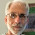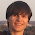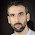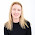Monday, September 26, 2016

Bayes's Theorem is not optional

Abstract: I present a probability puzzle, the Rain in Seattle Problem, and use it to explain differences between the Bayesian and frequentist interpretations of probability, and between Bayesian and frequentist statistical methods.  Since I am trying to clear up confusion, I try to describe the alternatives without commenting on their pros and cons.

Introduction

Conversations about Bayesian statistics sometimes get bogged down in confusion about two separate questions:

1) The Bayesian interpretation of probability, as opposed to the frequentist interpretation.

2) The Bayesian approach to statistical inference, as opposed to frequentist approach.

The first is a philosophical position about what probability means; the second is more like a practical recommendation about how to make inferences from data.  They are almost entirely separate questions; for example, you might prefer the Bayesian interpretation of probability by philosophical criteria, and then use frequentist statistics because of practical requirements; or the other way around.

Under the frequentist interpretation of probability, we can only talk about the probability of an event if we can model it as a subset of a sample space.  For example, we can talk about the probability of drawing a straight in poker because a straight is a well-defined subset of the sample space that contains all poker hands.  But by this interpretation, we could not assign a probability to the proposition that Hillary Clinton will win the election, unless we could model this event as a subset of all elections, somehow.

Under the Bayesian interpretation, a probability represents a degree of belief, so it is permissible to assign probabilities to events even if they are unique.  It is also permissible to use probability to represent uncertainty about non-random events.  For example, if you are uncertain about whether there is life on Mars, you could assign a probability to that proposition under the Bayesian interpretation.  Under the frequentist interpretation, there either is life on Mars or not; it is not a random event, so we can't assign a probability to it.

(I avoid saying things like "a Bayesian believes this" or "a Frequentist believes that".  These are philosophical positions, and we can discuss their consequences regardless of who believes what.)

In problems where the frequentist interpretation of probability applies, the Bayesian and frequentist interpretations yield the same answers.  The difference is that for some problems we get an answer under Bayesianism and no answer under frequentism.

Now, before I get into Bayesian and frequentist inference, let's look at an example.

The Rain in Seattle problem

Suppose you are interviewing for a data science job and you are asked this question (from glassdoor.com):
You're about to get on a plane to Seattle. You want to know if you should bring an umbrella. You call 3 random friends of yours who live there and ask each independently if it's raining. Each of your friends has a 2/3 chance of telling you the truth and a 1/3 chance of messing with you by lying. All 3 friends tell you that "Yes" it is raining. What is the probability that it's actually raining in Seattle?
Take a minute to think about it before you go on.  Then take a look at the responses on glassdoor.com. The top response, which uses Bayes's Theorem, is correct.  I'll explain the correct solution first; then I want to comment on some of the other responses.

The question asks you to compute the probability of rain conditioned on three yesses, which I'll write P(rain|YYY).

Now, here's an important point: you can't give a meaningful answer to this question unless you know P(rain), the probability of rain unconditioned on what your friends say.   To see why, consider two extreme cases:

1. If P(rain) is 1, it always rains in Seattle.  If your friends all tell you it's raining, you know that they are telling the truth, and that P(rain|YYY) is 1.

2. If P(rain) is 0, it never rains in Seattle, so you know your friends are lying and P(rain|YYY) = 0.

For values of P(rain) between 0 and 1, the answer could be any value between 0 and 1.  So if you see any response to this question that does not take into account P(rain), you can be sure that it is wrong (or coincidentally right based on an invalid argument).

But if we are given the base rate, we can solve the problem easily using Bayes's Rule,   According to the Western Regional Climate Center, from 1965-99 there was measurable rain in Seattle during 822 hours per year, which is about 10% of the time.

A base rate of 10% corresponds to prior odds of 1:9.  Each friend is twice as likely to tell the truth as to lie, so each friend contributes evidence in favor of rain with a likelihood ratio, or Bayes factor, of 2.  Multiplying the prior odds by the likelihood ratios yields posterior odds 8:9, which corresponds to probability 8/17, or 0.47.

And that is the unique correct answer to the question (provided that you accept the modeling assumptions).  More generally, if P(rain) = p, the conditional probability P(rain|YYY) is

Probability(8 Odds(p))

assuming that Odds() converts probabilities to odds and Probability() does the opposite.

Several of the responses on glassdoor.com provide what they call a frequentist or non-Bayes perspective:
Answer from a frequentist perspective:  Suppose there was one person. P(Y|rain) is twice (2/3 / 1/3) as likely as P(Y|no rain), so the P(rain) is 2/3.  If instead n people all say YES, then they are either all telling the truth, or all lying. The outcome that they are all telling the truth is (2/3)^n / (1/3)^n = 2^n as likely as the outcome that they are not. Thus P(YYY | rain) = 2^n / (2^n + 1) = 8/9 for n=3.  Notice that this corresponds exactly to the Bayesian answer when prior(raining) = 1/2.
And here's another:
I thought about this a little differently from a non-Bayes perspective.  It's raining if any ONE of the friends is telling the truth, because if they are telling the truth then it is raining. If all of them are lying, then it isn't raining because they told you that it was raining.  So what you want is the probability that any one person is telling the truth.  Which is simply 1-Pr(all lie) = 26/27.  Anyone let me know if I'm wrong here!
These are not actually frequentist responses.  For this problem, we get the same answer under Bayesianism and frequentism because:

1) Everything in this problem can be well-modeled by random processes.  There is a well-defined long-run probability of rain in Seattle, and we can model the friends' responses as independent random variables (at least according to the statement of the problem).

AND

2) There is nothing especially Bayesian about Bayes's Theorem!  Bayes's Theorem is an uncontroversial law of probability that is true under any interpretation of probability, and can be used for any kind of statistical inference.

The "non-Bayes" responses are not actually other perspectives; they are just incorrect.  Under frequentism, we would either accept the solution based on Bayes's Theorem or, under a strict interpretation, we might say that it is either raining in Seattle or not, and refuse to assign a probability.

Statistical inference is the process of inferring the properties of a population based on a sample.  For example, if you want to know the fraction of U.S. voters who intend to vote for Donald Trump, you could poll a sample of the population.  Then,

1) Using frequentist inference, you could compute an estimate of the fraction of the population that intends to vote for Trump (call it x), you could compute a confidence interval for the estimate, and you could compute a p-value based on a null-hypothesis like "x is 50%".  But if anyone asked "what's the probability that x is greater than 50%", you would not be able to answer that question.

2) Using Bayesian inference, you would start with some prior belief about x, use the polling data to update your belief, and produce a posterior distribution for x, which represents all possible values and their probabilities.  You could use the posterior distribution to compute estimates and intervals similar to the results of frequentist inference.  But if someone asked "what's the probability that x is greater than 50%", you could compute the answer easily.

So, how does this apply to the Rain in Seattle Problem?  It doesn't, because the Rain in Seattle problem has nothing to do with statistical inference.  It is a question about probability, not statistics. It has one correct answer under any interpretation of probability, regardless of your preferences for statistical inference.

Summary

1) Conversations about Bayesian methods will be improved if we distinguish two almost unrelated questions: the meaning of probability and the choice of inferential methods.

2) You don't have to be a Bayesian to use Bayes's Theorem.  Most probability problems, including the Rain in Seattle problem, have a single solution considered correct under any interpretation of probability and statistics.

UPDATE October 13, 2015:  A few people asked me how to solve this problem using Bayes's Theorem (based on probabilities) rather than Bayes's Rule (based on odds).  To apply Bayes's Theorem, you might find it helpful to use my "Bayesian update worksheet".  Here's one that shows how to solve this problem:

1.I was hoping to understand how to use Bayesian reasoning, but I was completely lost by the Bayesian argument. Would you mind elaborating the reasoning behind the segment of the post that starts at "A base rate of 10 ... " and ends at "Probability(8 Odds(p))". (I don't even understand how to read that final bit of notation!) Thanks.

1.my test blog has left a new comment on your post "Bayes's Theorem is not optional":

and subsitute 10% chance of rain for 25%, you should get the answer listed here:
0.1*(8/27) / ( 0.1*8/27 + 0.9*1/27 )
8/270 / 8/270 + 9/270
8/17
47.06%

In Downey's version, he uses both odds and probabilities, which makes the calculations in this case easier but, maybe, harder to follow.

Bayesian stats: you should estimate the prior probability that it's raining on any given day in Seattle. If you mention this or ask the interviewer will tell you to use 25%. Then it's straight-forward:

P(raining | Yes,Yes,Yes) = Prior(raining) * P(Yes,Yes,Yes | raining) / P(Yes, Yes, Yes)

P(Yes,Yes,Yes) = P(raining) * P(Yes,Yes,Yes | raining) + P(not-raining) * P(Yes,Yes,Yes | not-raining) = 0.25*(2/3)^3 + 0.75*(1/3)^3 = 0.25*(8/27) + 0.75*(1/27)

P(raining | Yes,Yes,Yes) = 0.25*(8/27) / ( 0.25*8/27 + 0.75*1/27 )

**Bonus points if you notice that you don't need a calculator since all the 27's cancel out and you can multiply top and bottom by 4.

P(training | Yes,Yes,Yes) = 8 / ( 8 + 3 ) = 8/11

But honestly, you're going to Seattle, so the answer should always be: "YES, I'm bringing an umbrella!"
(yeah yeah, unless your friends mess with you ALL the time ;)

Interview Candidate on Sep 12, 2013

2.Perhaps a more Frequentist-spirited answer would be to discuss the study design:

Your prior prob. of rain is under 50%, and in fact it's so low (at 10%) that *nothing* your 3 friends say could convince you the (posterior) prob. of rain is over 50%, even when they all agree it is raining.

In other words, your study has no power to change your mind! (from the prior decision that it's probably not raining.)

So why did you hassle your friends by asking them in the first place? Maybe that's why they lie to you 2/3 of the time :)

1.But refusing to answer the question is certainly in the spirit of frequentism.

2.[Allen, I didn't mean for my comment to sound snarky towards you! My cheekiness was directed at the hypothetical interviewer asking this question :) ]

Indeed, the question ends by asking for a probability. I totally agree with your solution to treating this as a toy probability puzzle. I agree there's no Bayes-vs-frequentist difference there. And I agree it's very important to distinguish Bayes' theorem from Bayesian inference.

On the other hand, the question *starts* with "You want to know if you should bring an umbrella."
Let's take this seriously as an interview question, meant to help the interviewer decide which candidates would bring the most value to the company.

What are they really getting at?
"We want to know if our company should take action U. It only makes sense to invest in performing U if there's at least 50% chance that R is true. We can (just barely) afford to run 3 expensive tests. Each test independently has 2/3 chance of correctly identifying whether R is true. If a sensible prior on U is 10%, and all 3 tests come back True, what will be the probability that R is indeed true?"

...then who would you rather hire?
Candidate A, who is content to stop after getting an answer of 47%?
Or Candidate B, who goes on to say:
"Look, even if all 3 tests agree in claiming that R is true, our estimated probability that R is true will *still* be under 50%. In all other cases, it'll be even lower. You're wasting money by running these 3 tests. If we can't afford more tests, let's just skip them and spend that money somewhere useful."

The spirit of frequentism is to step back from the given data and understand the operating characteristics of your statistical procedures---not just the analyses but the data-collection (design) too. It's a useful habit of mind, whether your final inferences/analyses end up Frequentist or Bayesian. And yep, sometimes that means refusing to answer a particular question, when that question isn't what's actually needed.

3.And I didn't mean to sound snarky towards you. Thanks for your comments!

3.two long related comments: 1) Why not use the priors for the particular days you will be visiting (I guess the chance of rain varies significantly over the year)? But then why not use as a prior the conditional probability of rain in Seattle given the atmospheric conditions at the moment? Or even, why not commission specific research to inform your prior: why stop at consulting the Western Regional Climate Center or the weather forecast? You can say - not worth it for the problem at hand, but this requires a separate analysis of how much effort it is worth spending on establishing a good prior for this problem. In our case, the answer is probably 'close to zero', as the costs of taking an umbrella are negligible. But then for somebody with zero knowledge about the weather in Seattle a flat prior of rain/no rain, or equivalently a frequentest analysis, would seem as justified as any other. 2) Which brings me to the second point. The problem is introduced as a decision-theoretic one (bring an umbrella or not) but then it asks for a probability that, however defined and computed, is not sufficient to answer the decision-theoretic motivating problem. And it seems to me that the added value of a Bayesian vs. a frequentist answer to the probability question cannot be demonstrated outside of a decision-theoretic setup in which the costs of establishing a prior are compared to the benefits of increased precision of the answer. (And you cannot just say, oh but everybody knows the prior chance of rain in Seattle is 0.5 or 0.1 or whatever, as this info is not provided in the set-up of the problem).

1.Thanks, Dimiter. You make some great points and I won't address them all, but I want to clarify one. The goal of my article is not to demonstrate the "added value of a Bayesian vs frequentist answer". The point I am trying to make is that the probability question, as stated, does not have two answers, one Bayesian and one frequentist answer. It has one answer that can be computed without any commitment to a Bayesian or frequentist interpretation of probability, and without any commitment to Bayesian or frequentist inference.

It does, as you point out, require the choice of a reference class, but that is a general difficulty with many probability problems; it is not a special difficulty for Bayesianism or frequentism.

4.Allen: I think the probability question, as stated, has not one and not two, but infinitely many answers. Why?
1) If we stick to the problem as stated, there is no information whatsoever that we can use to pick a prior probability of rain. So any prior must be as good as any other. By implication, that any posterior probability of rain can take any value between 0 and 1 (including these). So in the absence of any further information/ assumptions, the correct answers is probability of rain = [0;1]. We can say that the probability has significantly increased on hearing the signal from the friends, but we cannot see to how much, since we do not know the prior.
2) if we assume that if the probability was known with certainty in advance there would be no need for asking, we can exclude the boundaries of the interval, so p = (0, 1)
2b) if, in addition, we assume that probability is measured with finite precision (sey as whole percentages), the minimum prior probability becomes 0.01, so the interval for the posterior becomes p = (0.07, 1).
3) If we make the alternative assumption that the traveler would only ask for the solicited information if it would have a chance to change the decision (interpreted as moving the posterior above or below 0.5), the (posterior) probability becomes p = (0.5, 1). The friends send a signal that is as strong as it gets, so in the most adversarial prior it must just about move the probability above 0.5 so that in can satisfy the assumption of having a chance to influence the decision.
4) Now, if we want to make even further assumptions about what is known and what can be known in the framework of the problem, we can find reasons to pin the prior and, by implication the posterior, to any number within this range but a) this is not obviously warranted by the setup of the problem, and b) we enter the decision-theoretic problem mentioned in my previous post.
In sum, there might be one correct way to update probabilities based on prior information and new data. But in the absence of prior information and assumptions, any posterior probability must be correct.

5.Frankly, I have long thought of the bayesian approach as one that is best digested as a of morning joe. Frankly, the frequentists are more like a protein shake with shopped up celery and spinach.

However, non-parametric methods are the best. I love basic arithmetic.

Sir Thomas was a priest after all..

6.This comment has been removed by the author.

1.I deleted my initial comment because I was probably overthinking it. I grappled with your explanation and the other posts, but now understand your statement that only a Bayesian approach can lead to the right answer.

We want Prob(raining|YYY).
This is equal to Prob(raining and YYY)/Prob(YYY). We don't know Prob(raining and YYY).

However Prob(raining and YYY) also equals Prob(raining)*Prob(YYY|raining)

So we need Prob(raining)but don't know this. So as already mentioned by Dimiter, there are infinitely many answers in the absence of information.
However, we do know Prob(YYY|raining)=8/27

Furthermore,
Prob(YYY) = Prob(YYY|raining)*Prob(raining) + Prob(YYY|not raining)* Prob (not raining)

We also know Prob(YYY|not raining) = 1/27.

The frequentist solution is really a relative odds ratio: Prob(YYY|raining)/{ Prob(YYY|raining) + Prob(YYY|not raining)}. It is not really a probability at all.

Now when Prob(raining)=1/2 ( the best guess if you have no information) then the frequentist relative odds will equal the Bayesian probability.

If instead of Seattle the trip was to Los Angeles, and the Prob(raining) =0.01, then having three friends saying "Yes it's raining" will result in Prob(raining|YYY) =~0.075.

However if you asked 14 of your mostly truthful friends, and all 14 said,"yes dude, it's raining", then the conditional probability increase to ~0.993 (frequentist relative odds = ~0.99994).

This makes sense.

Thanks for posting this.

2.Hi Emile, I'm glad it is making sense.

But I want to clarify the point of my article. I am not saying that there are two answers to this question, one Bayesian and one frequentist, and the Bayesian one is right.

I am saying that there is only one answer to this question, and it is neither Bayesian nor frequentist. It is just a consequence of the laws of probability.

The issue Dimiter raised is called the reference class problem: https://en.wikipedia.org/wiki/Reference_class_problem

And while the reference class problem is relevant to the problem, it is a general problem for all of probability, and not specifically Bayesian or frequentist, either.

7.Hello Allen, I'm having difficulty solving this problem using Bayes Theorem but have no idea where I'm going wrong. Could you please shed me a light?

We have:

P(rain|YYY) = P(YYY|rain)*P(rain) / P(YYY)

P(YYY|rain) = 8/27 ?
P(rain) = 0.1
P(YYY) = 8/27 ?

Are the previous values correct? If they are then P(rain|YYY) = 0.1*8/27 / 8/27 = 0.1

This would imply that P(rain|YYY) is not dependent on P(YYY) at all. What am I doing wrong?

1.Hi. I just added a worksheet that shows how to solve this problem using Bayes's Theorem.

8.Hi Allen,

Thank you for posting this problem. It seems very interesting. I have a quick question: why do we compute the probability of YYY? This information is already given in the problem ("All 3 friends tell you that "Yes" it is raining."), so P{YYY} should be 1?

Thank you!

9.I wonder if you are still following and interested in this thread.

I was trying to map the example into other examples---eg you have a city of people and take a sample of 1, 2, and then 3 and test them for drugs . the drug test is only 2/3rds accurate ( if they have drugs in their system then 2/3rds of the time the test says Yes they do, and 1/3rd No they don't . Suimialrily if they dont have drugs in them, then 2/3rds of the time they come out 'negative', and 1/3rd positive.

You can consider cases like 'maybe i dont know the size of the city--is it 1, 2, 3 or a million people'? To apply Bayes theorem you might need a 'prior' like what % of people in that city are on drugs.

I'm really interested in a 'recursion' so you can go from 1 sample response (in your example, one friend), to 2, to 3, etc.

I actually think of this in terms of the ising model and spin glasses of statistical physics.

Also in term s of Venn diagrams--in you case the big circle is Seattle, and its cut in 2 by a line saying on one side of the circle (CITY) its raining and other side its not. Then you sample somone in the city but you dont know if they are on the rainy or sunny side, and also you only know they are telling the truth 2/3rds of the time when you ask them if its raining. (or you could ask them if they are on drugs, or did the crime.)

m impression is bayesianism reduced to frequentism if P(Rain) is unknown (just 50/50).
also if you ask 3 people do you use (1/3rd +1/3rd +1/3rd)/3 or (1/3)^3 (times probabilities) . i think those are 2 different slightly different assumptions---one independent random variables (multiply) versus additive --which reduces to same thing sometimes.

i just view bayes theorem or rule as a version of the definition of conditional probability.